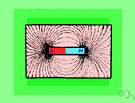# magnetic field strength

Also found in: Thesaurus, Medical, Encyclopedia, Wikipedia.
Related to magnetic field strength: magnetic flux density

## magnetic field strength

n.
Magnetic intensity.
ThesaurusAntonymsRelated WordsSynonymsLegend:
 Noun 1magnetic field strength - the amount of magnetic flux in a unit area perpendicular to the direction of magnetic flowfield intensity, field strength - the vector sum of all the forces exerted by an electrical or magnetic field (on a unit mass or unit charge or unit magnetic pole) at a given point in the field
References in periodicals archive ?
Based on the type, the magnetoresistive sensors segment is likely to have a huge demand because these sensors have a wide operating range and are commonly used to detect magnetic field strength.
Caption: Top: The total magnetic field strength over the nearside of the Moon compiled from Lunar Prospector and Kaguya data reveals a large "hole" in the northwestern quadrant.
In order to produce magnetic field strength measurements that would allow a wide range of students to be successful with this task, a set of prewrapped solenoids was designed and calibrated.
Earth's magnetic field strength has declined by about 10 percent over the last century and if such rapid flips are any indication, something similar could happen in the future, too.
where [[mu].sub.0] = 4[pi] x [10.sup.-7] H/m is the magnetic constant; [H.sub.S] is the amplitude of the tangential component of the magnetic field strength on the surface of the stator back, A/m; x [R.sub.S] is the outer radius of the stator core back, m; [tau] is the pole division, m; [R.sub.k] is the inner radius of the stator case, m; 2p is the number of poles.
The darkness of the umbrae is generally correlated with the magnetic field strength. Hence, the strongest magnetic field in each sunspot is located in the umbra in most cases.
When magnetic fluid is exposed to a rotational magnetic field, a vector of magnetic field strength can be divided into x and y components, [H.sub.x] and [H.sub.y], and also the magnetization components [??] can be separated into the x and y directions and calculated by the following equation:
The company said the Magnetom Terra is the first 7T MRI system is cleared for clinical use in the US, more than doubling the static magnetic field strength available for use in the nation.
Both models show that an increase in magnetic field strength results in an increase in stable operating voltage and a decrease in the maximum deflection of the membrane.
1 and described as following; (a) magnetization: the magnetic field strength is increased causing magnetic entropy to decrease which further increases MCM's temperature; (b) cold blow: some heat transfer fluid is employed to absorb MCM heat to cool it down to ambient temperature; (c) demagnetization: the magnetic field intensity is decreased causing magnetic entropy to increase which further lowers MCM's temperature; (d) hot blow: again a heat transfer fluid is used to reject the heat to MCM increasing its temperature and thus the cycle goes on.
(Note: belt speed, belt width, capacity, and bulk density all are factors in calculating the material burden depth.) The burden depth determines the suspension height of the magnet and consequently the effective magnetic field strength at the belt surface.
Clearly, testing at one magnetic field strength could distinguish between two or perhaps three types of materials, but determining which parts were good or bad is likely to be inconclusive.

Site: Follow: Share:
Open / Close